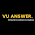# MTH101 Quiz 1 2022 Solved - Calculus and Analytical Geometry Quiz Solved

VU Answer Provide you to Prepare your Online Test of MTH101 Quiz 1 2022 Solved. Calculus and Analytical Geometry Quiz Questions and Answers. Download MTH101 Quiz 1 Solved PDF.

MTH101 Quiz 1 2022

Calculus and Analytical Geometry Quiz Question and Answer

1.  If f(x) x^3 +1, then which of the following is not True about it. The Slope of tangent line at x =1 is 3

2. If f(x) = ax+bx+c, then its derivatives with respective to x is……. a+b

3. The Equation of tangent line at Point (x0, y0) is y+y0=m tan(x+x0) False

4. Derivative of Graph of Straight line Bisecting the First and Third Quadrants is….  +1

5. What is Derivative of 7x3   21x2

6. Derivative of Straight line of Parallel to y-axis is……. Zero

7. When the Slope of the Tangent line Approaches to ± infinity, then…. Occurs Circle

8. To Find the Slope of Tangent Line at a Particular point along a Curve, you Have to Calculate the ………………… Instantaneous Velocity

9. Average Velocity is Obtained by Dividing the………. Total Distance by Time Elapsed

10. If f(x) = cos(2x) then its derivates By Chain Rules is ……… -Sin(2x).2

11. What is Derivatives of 101? 0

12. The Derivative of y=cosecx at x=0 is……. Infinity

13. If f(x) = x5 and g(x) = x2 , Then the Derivative of F(x)/g(x)  is……… 3x2

14. The Derivative of sinx.cosx at x=0 is…....... 1

15. If f(x) = 2x+2 and g(x) = x2 + 3x, the Derivative of f(x) + g(x) is……. 5 + 2x

16. The Instantaneous rate of Change of y^2+4 with Respect to x = 2 is …… 4

17. What is Derivative of 2x2 sin(x5)? None of these

18. What is Derivative of Sin (20x)? 20cos(20x)

19. For the Curve f(t) = -2t+3, the Instantaneous Rate of Change of ‘f(t)’ at t = 1 is -2

20. Let f(x) = Cos(x)/6, the Derivative of f(x) is……. –Sinx/6

21. The Sum of Two Differentiable Functions is Always Differentiable is …. True

22. Derivative of Cos 4x By Using Chain Rule is……… -4Sin 4x

23. What is The Derivative of Sin(x) at x = Sin(x) at x = π/2?          1

24. If Y=x+1 then Average rate of Change of ‘y’ w.r.t ‘x’ Over the Internal [ 1,5,2,5] will be…… 1

25. If the Function is Differential at a Point, then it is also _______ at the Point. Continuous

26. What is the Derivative 3cot 2(x)……? -6cosecx2(2x)

27. If y = 5x + bx – c, then Derivative w.r.t x is……… 5 + b

28. Let f(x) = cos(x)/6 the Derivative of f(x) is…. –Sinx/6

29.  The Function of f(x) = f(x) is Differential at x=0………………... False

30. Let Y = x^2+1 Then the Instantaneous rate of Change y with respect to x at point -2 is 3

31. A Function f(x) is Differentiable on (a,b) if is Differentiable at……..point in (a,b)…… Each

32. What is the Derivative of Sin(x) + Cos(x)? Cos(x) + Sin(x)

33. (f,g)’ = f,.g- f.g  False

34. Velocity is the rate of change of position w.r.t……… Time

35. What is the Derivative of 39x 14/13 42x 1/33

36. Point slope from the tangent line is y-3=6(x-3) y=6x-15

37. What is the Derivative of 2x+3x2…... 4x+3

38. If f(x) = cot(2x), then the Derivative of f(x) is…….  -2csc2(2x)

39. If f(x) = sec(x) and g(x) = tan(2x), then the Derivative of f(x) – g(x) is……… sec(x) tan(x) – 2sec2(2x)

40. If f(x) = Sin4(x2), then the Derivative of f(x) is………... None of these

41. A Differentiable Functions is always Continuous True

Thanks you for reaching us.

If you found any mistake or help tell us in comment section.

MTH101 Quiz 1 Solved

Calculus and Analytical Geometry Quiz Solved

Calculus and Analytical Geometry MCQS

1.1.